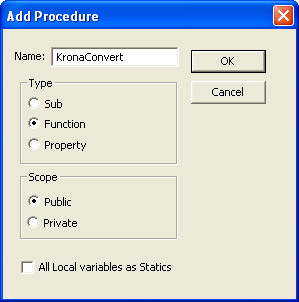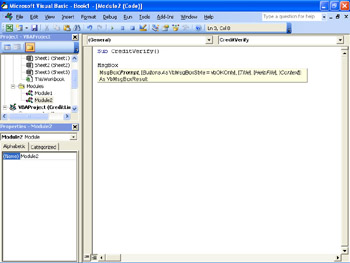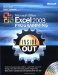# Defining Function Procedures

 < Day Day Up >

A Function procedure is similar to a Sub procedure, but a function can also return a value. A Function procedure can take arguments, such as constants, variables, or expressions that are passed to it by a calling procedure. As an example, consider the SUM function you most likely use all the time when you create formulas in an Excel worksheet. The following example formula finds the sum of the values in cells C14 to H14, J14 and adds 100:

=SUM(C14:H14, J14, 100)

The SUM function adds everything in the statement that calls it; if it can’t make sense of its input, such as when it tries to add a non-numerical value, it will return an error message and display an error code. If a Function procedure has no arguments, its Function statement must include an empty set of parentheses.

`[Private | Public] [Static] Function name [(arglist)] [As type][statements][name = expression][Exit Function] [statements][name = expression] End Function `

Table 5-2 describes the elements of a Function procedure, many of which will be familiar from the description of a Sub procedure.

Table 5-2: Elements of a Function Procedure

Element

Description

Public

An optional element that indicates the Function procedure is accessible to all other procedures in all modules. If used in a module that contains an Option Private statement, the procedure is not available outside the project.

Private

An optional element that indicates the Function procedure is accessible only to other procedures in the module where it is declared.

Static

An optional element that indicates the Function procedure’s local variables are preserved between calls. The Static attribute doesn’t affect variables that are declared outside the Function procedure, even if they are used in the procedure.

name

A required element that indicates the name of the Function (for example, Function InterestDue). The name does need to follow the standard variable naming conventions.

arglist

An optional list of variables representing arguments that are passed to the Function procedure when it is called. Multiple variables are separated by commas.

type

An optional statement that specifies the data type of the result returned by the Function procedure. For example, a function returning an integer value would have As Integer in this space.

statements

An optional group of statements to be executed within the Function procedure.

### Creating a Function Procedure

You can create a Function procedure in an existing code module by opening the module in the Visual Basic Editor by typing the following:

`Function name()…End Function `

You will need to name the function and put instructions in the middle where the ellipsis is now, but you can do it much more quickly using the Add Procedure dialog box.

To add a Function procedure to a code module, follow these steps:

1. Click Tools, Macro, Visual Basic Editor to display the Visual Basic Editor.

2. If necessary, click Insert, Module to create a new code module (or if you want to create a new module for organizational purposes).

3. Click Insert, Procedure to display the Add Procedure dialog box.

4. Type the name of your procedure in the Name box.

5. Select the Function option button.6. Click OK.

 Note As with Sub procedures, you can make your Function procedures available to procedures in every other workbook by putting the Public keyword in front of the declaration. The Public keyword is added by default when you add a procedure using the Insert Procedure dialog box.

### Running Function Procedures

If you want to run a Function procedure, you can do so using one of the following methods:

• Use the Function procedure in a formula.

• Call the Function procedure from within another procedure.

• Call the Function procedure from a cell on a worksheet.

 Important Your Function procedures don’t appear in the Macros dialog box.

You’ve already seen the first technique several times in the preceding two chapters, and you’ve no doubt used functions many times in your worksheets. One example of an existing function you might use in a worksheet would be =NOW(), which returns the current date and time. The third way to run a Function procedure is to call it from a cell on a worksheet. To do so, you can call it the same way you would call any other function (for example, =Amortize(ActiveCell.Value)).

So, when might you want to use a function procedure instead of a Sub procedure to operate on a value? There are two such times: when you want to use the result of the function in an expression in your VBA code, or when you want to use the result in a formula in one of your worksheets. For example, if The Garden Company repackaged potting soil from 25-pound bags into 5-pound bags, you could create a function that multiplied the number of 25-pound bags by five to generate the total number of small bags. Then you could create a function such as this one:

`Function SmallBags(intLargeBags as Integer) as Integer    SmallBags = intLargeBags * 5End Function`

Once created, you could call the function from within a cell using the formula =SmallBags(C16) to convert the number of large bags of potting soil in an order, which was stored in cell C16, into the number of small bags of potting soil that order will produce.

### Passing Arguments to Procedures

So far in this chapter, you’ve seen procedures that operate on fixed values, such as the contents of a cell, and procedures that don’t operate on any values at all, such as the NOW function. When you write a procedure that operates on a value from a cell by calling the cell’s value from inside the procedure using the ActiveCell.Value property or the Range(<cell>).Value property, you don’t need to worry about passing values from variables. Unfortunately, the situation won’t always be so straightforward. There might be times where you want to operate on a value that’s stored in a variable in a procedure, not in a worksheet cell. That’s when you need to tell the procedure the values on which you want it to operate; those values are called arguments.

You probably noticed that the first line of the SmallBags function looked different from most of the other procedures you’d seen earlier in the chapter. The following function, which recommends a retail price of an item that’s 180 percent of the item’s wholesale price, also takes an argument:

`Function MarkupPrice(curItemPrice as Currency) as Currency    MarkupPrice = curItemPrice * 1.8End Function`

Let’s take a moment to break down the first line in the function. The Function keyword is familiar, as is the function name that follows it, but the next two elements are new. The element in the parentheses, curItemPrice as Currency, is the name and data type of the variable that’s being passed to the function. In other words, regardless of how the function gets its variable, it knows to treat the value it receives as a variable of type Currency.

 Caution As with other functions, if the data the function receives is of the incorrect type, the function will return a #VALUE! error message in the worksheet cell where the function is called.

The last element of the first Function procedure statement is the second occurrence of as Currency. That element tells the function the data type of the value it returns to the formula or procedure that called it. Most of the time the result of the procedure will be returned as the same data type as the value passed to the procedure, but you might want to divide a single by an integer and return an integer value. One situation where that would be the case would be if you have 22.3 pounds of potting soil and want to see how many full 5-pound bags you could make out of it.

So where is the value calculated by the procedure stored? It’s stored in a variable with the same name as the Function procedure. In the code listed earlier, the second line executes the arithmetic.

`    MarkupPrice = intLargeBags * 1.8`

The MarkupPrice variable is created using the data type named at the end of the first statement in the procedure.

If you need to pass more than one argument to a procedure, you do so by separating the arguments by commas, as in the following example:

`Function ConvertMultiple (sngKrona as Single, sngEuro as Single) as Single `Inside Out
Protecting Your Original Cell Data

One of the dangers of programming is that you can inadvertently change the original values in your worksheet. For example, if you create a Sub procedure that assigns some value to the active cell, you’ll end up destroying your original data. So, in addition to always creating backup copies of all your data, you can consider using the ByVal keyword to have the procedure use a copy of the data and not the original cell value (or array, or object, or whatever) itself. A Sub procedure to calculate the number of small bags of soil to be created from a number of large bags would be written the following way:

`Sub SmallBags(byVal intLargeBags)    MsgBox("The number of large bags is " & intLargeBags * 5).End Sub`### Passing Named Arguments

All the procedures in this chapter that have called a procedure have passed the arguments the procedure requires in an order the procedure expects. For example, when you type MsgBox to begin a statement to create a message box, the Visual Basic Editor displays a ToolTip indicating the expected arguments, as shown in Figure 5-5.Figure 5-5: The Visual Basic Editor helps you create effective procedures by listing the expected arguments.

If you want to make the arguments you pass to a procedure easier to read, if a bit more verbose, you can use named arguments. A named argument consists of the name of the argument followed by a colon and an equal sign (:=) and the value assigned to the argument. For example, the MsgBox procedure has the following syntax:

`MsgBox(prompt[, buttons] [, title] [, helpfile, context])`

If you wanted to create a message box with a specific title and prompt (two of the arguments listed earlier), you could do so with the following statement:

`MsgBox Title:="Status Report", Prompt:="Order Accepted"`

For more information on creating message boxes, see Chapter 4, “VBA Programming Starter Kit.”

 < Day Day Up >Microsoft Office Excel 2003 Programming Inside Out (Inside Out (Microsoft))
ISBN: 0735619859
EAN: 2147483647
Year: 2006
Pages: 161

Similar book on Amazon

flylib.com © 2008-2017.
If you may any questions please contact us: flylib@qtcs.net